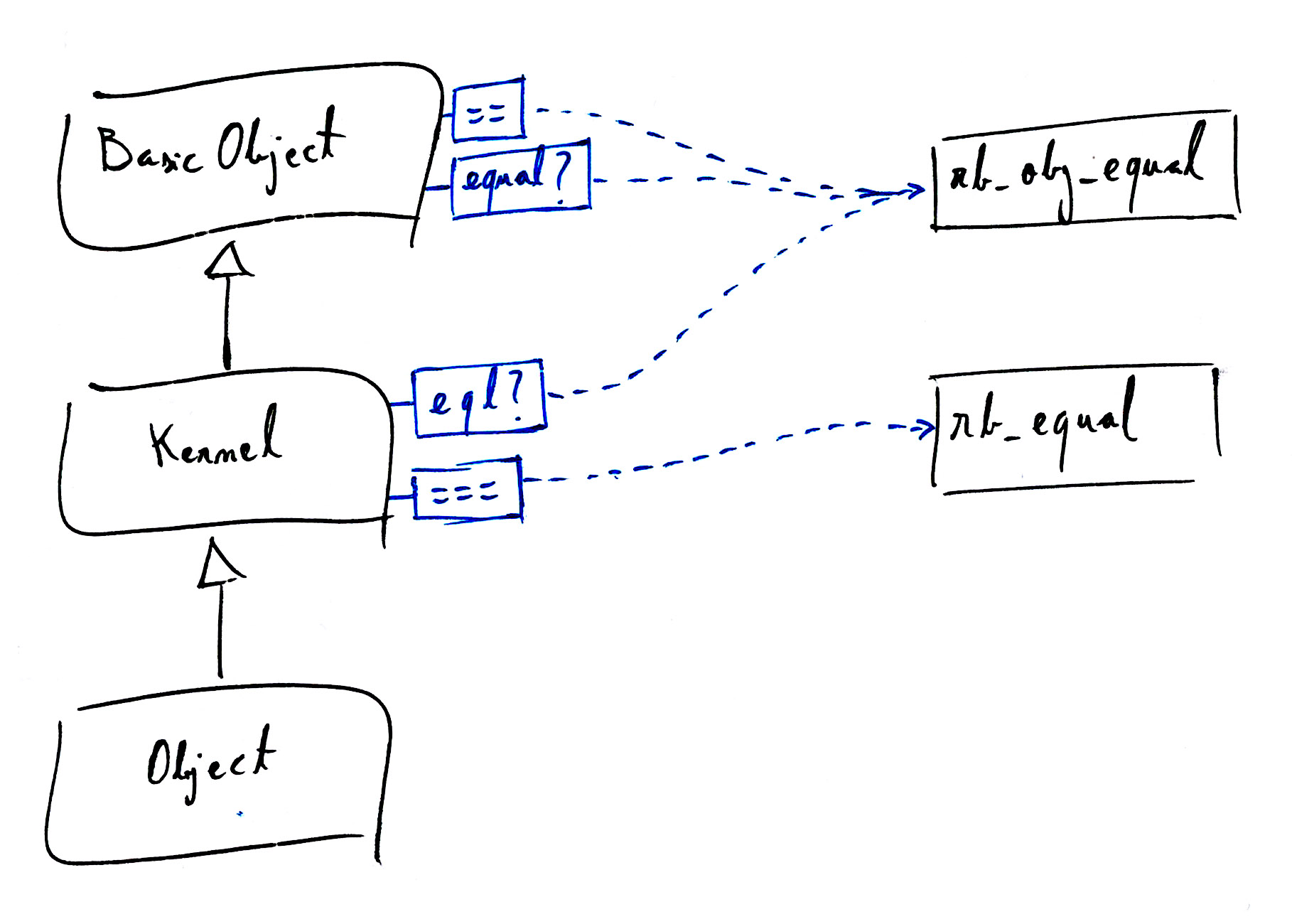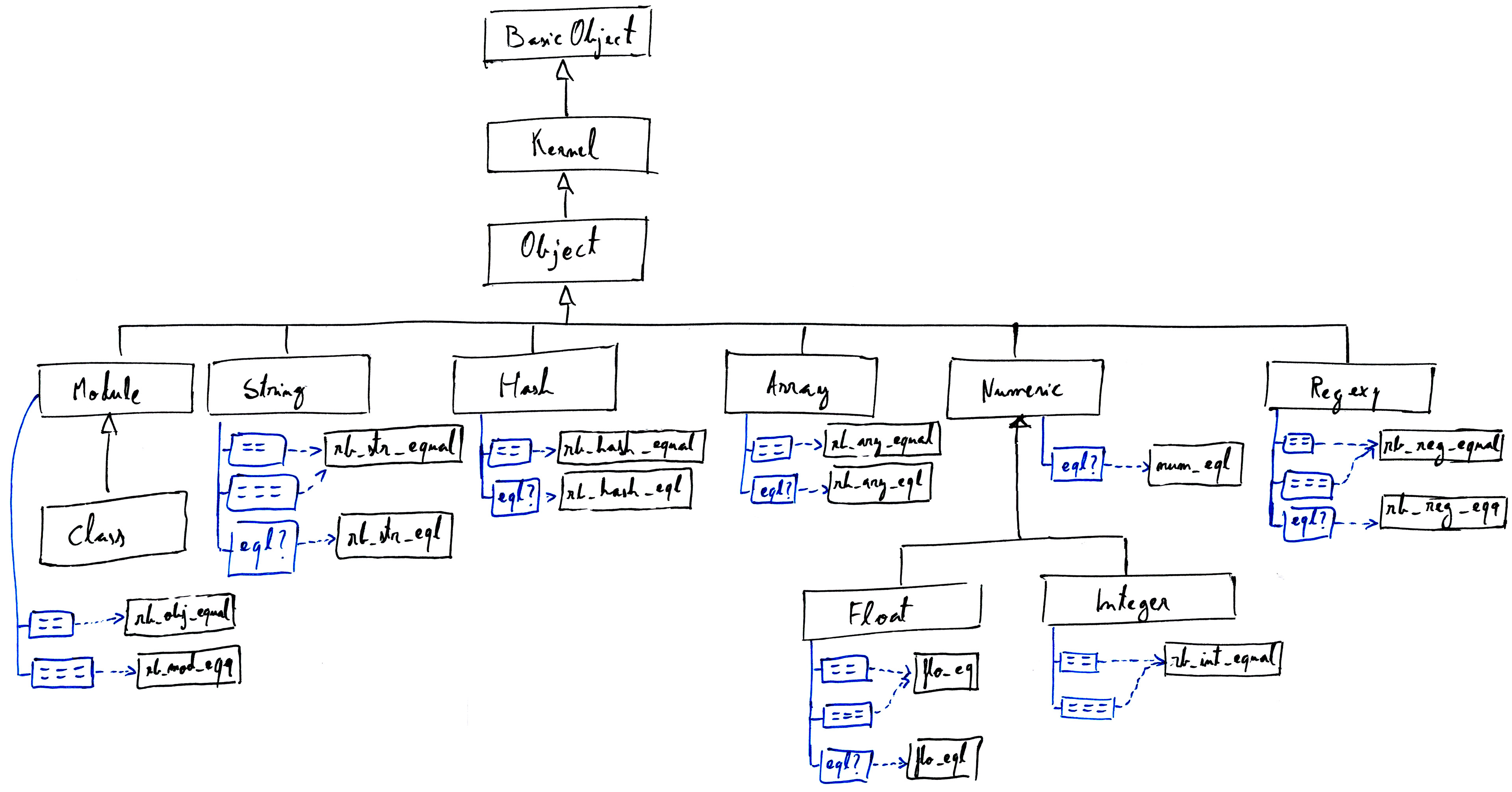# Four almost equal methods

Let’s explore the different ways we can compare objects in Ruby. We’ll investigate the implementation of the following:

• Equality, or ==.
• Case Equality, or ===.
• eql?
• equal?

For conciseness, I’ve decided to leave out the following ones, maybe will be covered in a different post:

• Pattern Match, or =~; and its opposite, !~.
• instance_of?, kind_of? & is_a?.
• The spaceship operator, or <=>.

## Diving into Ruby internals

In object.c, we can find:

rb_define_method(rb_cBasicObject, "==", rb_obj_equal, 1);
rb_define_method(rb_cBasicObject, "equal?", rb_obj_equal, 1);


A few lines below we can also find this:

rb_define_method(rb_mKernel, "eql?", rb_obj_equal, 1);


So the three methods ==, equal? & eql? map to the same C function: rb_obj_equal.

The case equality however is mapped to rb_equal instead:

rb_define_method(rb_mKernel, "===", rb_equal, 1);


Some methods are bound to BasicObject and others are bound to Kernel. But that doesn’t matter too much as almost all other classes inherit from both. Let’s see how the look up chain would look so far:At this stage, I find it interesting that Ruby has 3 different methods mapped to the same function. This is partly explained in the official documentation, it says that the equality operator is expected to be overriden by descendants, whereas the equal? should never be overriden.

Not only the three method names map to the same function, but also rb_equal may end up calling rb_obj_equal too:

VALUE
rb_equal(VALUE obj1, VALUE obj2)
{
VALUE result;

if (obj1 == obj2) return Qtrue;
result = rb_funcall(obj1, id_eq, 1, obj2);
if (RTEST(result)) return Qtrue;
return Qfalse;
}


That function means:

• If obj1 == obj2 (in its C meaning), then return true.
• Otherwise, rely on the object’s implementation of ==. Ie. obj1.==(obj2).

As we saw earlier, the default implementation of == is bound to rb_obj_equal so === will end up invoking rb_obj_equal in many cases.

Let’s now see where this all seems to be converging to, the definition of rb_obj_equal:

VALUE
rb_obj_equal(VALUE obj1, VALUE obj2)
{
if (obj1 == obj2) return Qtrue;
return Qfalse;
}


At Object level, all four methods boil down to that simple value comparison at C level (or an equivalent one in rb_equal). But we still don’t know why so many different names are needed.

That’s because so far we’ve only looked at the core classes. If we zoom out we’ll see how each method has a different meaning in the context of a subclass.

For example, is 1 equal to 1.0? Depends on what you consider equal:

• They have the same numeric value, so they’re equal.
1 == 1.0
# => true

• They’re objects of different type, so they’re not.
1.eql? 1.0
# => false


## The big picture

I won’t go into detail with all subclasses because it’ll be an endless post. But I do want to share the following diagram where you can see what classes implement a refinement of one of these methods.I’ve also included the name the internal C function that implements each method.

### The case equality

One interesting thing to notice is that Module and Regexp have a definition of the case equality ===.

Because the case statement internally will call === to select the apropriate execution branch we can do things like the following:

case "github.es"
when /(.+).com$/ puts "It's a .com" when /(.+).es$/
puts "It's a .es" # <-- chosen branch
end

# Because:
/(.+).es\$/ === "github.es" # => true


And:

case 3.0
when Integer
puts "It's an integer!"
when Float
puts "It's a float!" # <-- chosen branch
end

# Because:
Float === 3.0 # => true


## Conclusions

In summary:

• equal? is never overriden, therefore it’ll always map to that == comparison at C level.
• The equality operator == is overriden by all subclasses to indicate if two objects are equal or not, whatever that means in the context of that subclass.
• eql? is overriden by some subclasses to implement a custom kind of equality, like the example above with Numeric.
• The case equality operator === is overriden by very few subclasses mainly with the purpose of making it easy to access from a case statement.

### Want to know more?

I’m leaving it here for now, this should be good enough to get an idea of how comparisons are implemented in the core of the language. If you want to dig deeper, I hope the diagram is useful as a reference guide to browse the source code.

Another way to satisfy your curiosity is by leaving your question in the comments, I hope you enjoyed the journey!